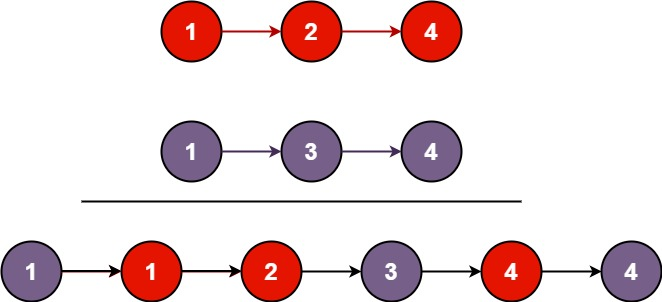# 21. Merge Two Sorted Lists

Easy
You are given the heads of two sorted linked lists `list1` and `list2`.
Merge the two lists in a one sorted list. The list should be made by splicing together the nodes of the first two lists.
Example 1:Input: list1 = [1,2,4], list2 = [1,3,4]
Output:
[1,1,2,3,4,4]
Example 2:
Input: list1 = [], list2 = []
Output:
[]
Example 3:
Input: list1 = [], list2 = 
Output:

Constraints:
• The number of nodes in both lists is in the range `[0, 50]`.
• `-100 <= Node.val <= 100`
• Both `list1` and `list2` are sorted in non-decreasing order.

### 解題

/**
* type ListNode struct {
* Val int
* Next *ListNode
* }
*/
func mergeTwoLists(list1 *ListNode, list2 *ListNode) *ListNode {
cur1 := list1
cur2 := list2
for cur1 != nil || cur2 != nil {
if cur1 == nil {
cur.Next = cur2
cur = cur.Next
cur2 = cur2.Next
} else if cur2 == nil {
cur.Next = cur1
cur = cur.Next
cur1 = cur1.Next
} else {
if cur1.Val <= cur2.Val {
cur.Next = cur1
cur = cur.Next
cur1 = cur1.Next
} else {
cur.Next = cur2
cur = cur.Next
cur2 = cur2.Next
}
}
}